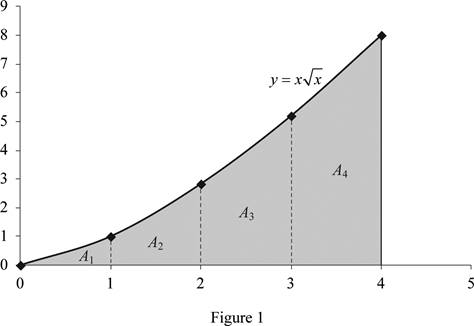# The value of the area under the curve.### Single Variable Calculus: Concepts...

4th Edition
James Stewart
Publisher: Cengage Learning
ISBN: 9781337687805### Single Variable Calculus: Concepts...

4th Edition
James Stewart
Publisher: Cengage Learning
ISBN: 9781337687805

#### Solutions

Chapter 5, Problem 37RE
To determine

## The value of the area under the curve.

Expert Solution

The area under the curve is 12.8.

### Explanation of Solution

Given information:

The equation of curve is y=xx (1)

The region lies between x=0 and x=4.

Calculation:

To find the value of y by using Equation (1) as shown below:

Substitute 0 for x in Equation (1).

y=00=0

Substitute 1 for x in Equation (1).

y=11=1

Similarly calculate the values of y for different x values as shown in Table (1).

 x y 0 0 1 1.00 2 2.83 3 5.20 4 8.00

Plot the curve using the values from Table (1).

Draw the diagram for the equation of curve as shown in Figure (1).Refer Figure (1)

Here, area of the shaded portion 1 is A1, area of the shaded portion 2 is A2, area of the shaded portion 3 is A3 and the area of the shaded portion 4 is A4,

To find the area under the graph using Figure (1) as shown below:

Area=A1+A2+A3+A4=(12×1×1)+(12×1×(1+2.83))+(12×1×(2.83+5.2))+(12×1×(5.2+8))=0.5+1.915+4.015+6.6=13.03

Therefore, the rough estimate of area under the graph is 13.03.

The expression to find the area under the curve as shown below:

04xxdx=04x3/2dx=(x32+132+1)04=(x5/252)04=25(45/20)

04xxdx=25×32=645=12.8

Therefore, the area under the curve is 12.8.

### Have a homework question?

Subscribe to bartleby learn! Ask subject matter experts 30 homework questions each month. Plus, you’ll have access to millions of step-by-step textbook answers!